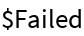#Function Repository Resource:

# CompleteSquare

Algebraically rewrites an expression by completing the square

Contributed by: Paco Jain (Wolfram Research)
 ResourceFunction["CompleteSquare"][expr,x] returns an expression equivalent to expr, when possible, of the form (a x+b)^2 + c where a,b, and c are FreeQ of x.

## Details and Options

If no expression of the required form can be found, CompleteSquare returns \$Failed:

## Examples

### Basic Examples

Complete the square of a quadratic:

 In:=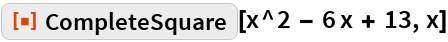Out=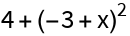Complete the square of a quadratic with a mixture of numeric and symbolic coefficients :

 In:=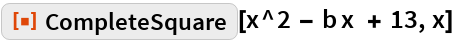Out=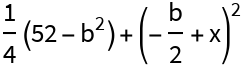Complete the square of the generic quadratic:

 In:=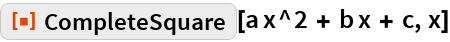Out=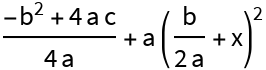Complete the square of a non-quadratic, even-ordered polynomial:

 In:=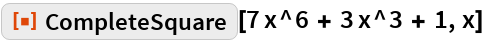Out=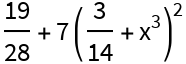### Properties and Relations

If no expression of the required form can be found, CompleteSquare returns \$Failed:

 In:=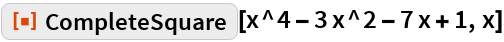Out=